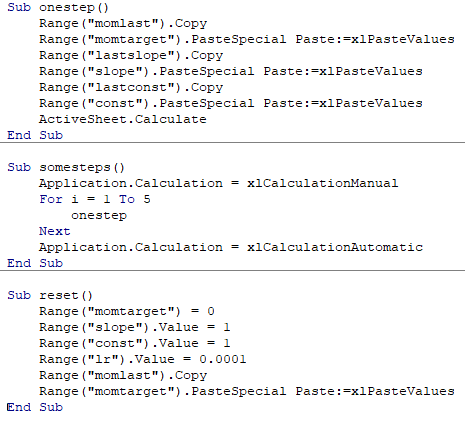# Lesson 4 Where are the first value of momentum multiply come from(graddesc.xlsm, momentum page)

Lesson 4, 32.44min

My problems are

1: Where are the values, -19.28 and 162.84 come from?Are they some sort of random values?
2: 0.9 is momentum, so what are 0.1?What is it for
3: Purpose of momentum is setting how important of last few average be?

I upload an image, hope this could make this question easier to understand.

Just to be accurate, Lesson 4, 32:44 has -18.33 and 98.25 in J3 and K3 respectively.
The values -19.28 and 162.84 are generated only after the first Run (or 5 epochs).

Now here is how I see it:

The values in J3 and K3 are copied from J33 and K33 respectively. And the values in J33 and K33 are copied from J32 and K32.

The confusing bit perhaps is that you don’t see J32 and J33 or K32 and K33 containing the same values. This is because the moment the Run macro copies values from J32 to J33 and K 32 to K33, the whole worksheet is automatically recalculated, so J32 and K32 end up with brand new values from the latest Run.

In case you’re wondering what are the initial values for J3 and K3, they are both zeros. To prove that you can just enter 0 in J33 and 0 in K33 and the worksheet will recalculate J32 and K32 to be -18.33 and 98.25 respectively.

And of course another excellent proof are Jeremy’s momentum-worksheet macros that do all that magicWhen it comes to the 0.9 and 0.1 question, I think about it as the weighting to use for the average derivative and the current derivative when calculating the “total” derivative to use for the current step. I.e. with 0.9 and 0.1 we tell the algorithm to add up 90% from the average derivative with only 10% from the current step derivative.

You will notice that if you change J1 from 0.9 to 0.7, the value in K1 changes to 0.3 (the two (J1+K1) always add up to 1.0 (or 100%).

So changing 0.9 to 0.7 tells the algorithm to calculate the “total” gradient by adding a 30% contribution from the current step gradient and 70% contribution from the momentum (average) gradient.

And that’s the way I see it.

6 Likes